https://doi.org/10.5194/angeo-41-129-2023
https://doi.org/10.5194/angeo-41-129-2023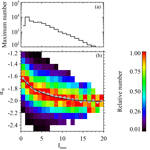# Effect of intermittent structures on the spectral index of the magnetic field in the slow solar wind

Xin Wang, Xuanhao Fan, Yuxin Wang, Honghong Wu, and Lei Zhang
Abstract

Intermittent structures are ubiquitous in the solar wind turbulence, and they can significantly affect the power spectral index (which reflects the cascading process of the turbulence) of magnetic field fluctuations. However, to date, an analytical relationship between the intermittency level and the magnetic spectral index has not been shown. Here, we present the continuous variation in the magnetic spectral index in the inertial range as a function of the intermittency level. Using the measurements from the Wind spacecraft, we find 42 272 intervals with different levels of intermittency and with a duration of 5–6 min from 46 slow-wind streams between 2005 and 2013. Among them, each of the intermittent intervals is composed of one dominant intermittent structure and background turbulent fluctuations. For each interval, a magnetic spectral index αB is determined for the Fourier spectrum of the magnetic field fluctuations in the inertial range between 0.01 and 0.3 Hz. A parameter Imax, which corresponds to the maximum of the trace of the partial variance increments of the intermittent structure, is introduced as an indicator of the intermittency level. Our statistical result shows that, as Imax increases from 0 to 20, the magnetic spectrum becomes gradually steeper and the magnetic spectral index αB decreases from −1.63 to −2.01. Accordingly, for the first time, an empirical relation is established between αB and Imax: ${\mathit{\alpha }}_{B}=\mathrm{0.4}\mathrm{exp}\left(-{I}_{\mathrm{max}}/\mathrm{5}\right)-\mathrm{2.02}$. The result will help us to uncover more details about the contributions of the intermittent structures to the magnetic power spectra and, furthermore, about the physical nature of the energy cascade taking place in the solar wind. It will also help to improve turbulence theories that contain intermittent structures.

Share
Dates
1 Introduction

Intermittent structures are ubiquitous in the solar wind turbulence. They correspond to the long tail of the non-Gaussian probability distribution functions of plasma or field fluctuations . Previous studies have revealed that intermittent structures are associated with current sheets and different types of discontinuities at small scales (tens of seconds) as well as with the boundary between two adjacent flux ropes at large scales (tens of minutes) in the solar wind turbulence. These structures play an important role in the turbulence cascading and dissipation processes (; ; and the references therein).

Intermittent structures with large-amplitude fluctuations make a substantial contribution to the shape and power level of magnetic field spectra, which are directly related to the physical nature of the energy cascade taking place in the solar wind . They often make the magnetic spectra become steeper . In previous studies, time series of discontinuities have been reported to produce an f−2 energy spectrum in the inertial range. Moreover, more recently, it has been found that the discontinuities can also produce magnetic power spectra that are shallower than f−2. studied the effect of current sheets on the magnetic power spectrum from Ulysses observations. They found that the abundant-current-sheet periods and current-sheet-free periods show ${f}^{-\mathrm{5}/\mathrm{3}}$ Kolmogorov scaling and ${f}^{-\mathrm{3}/\mathrm{2}}$ Iroshnikov–Kraichnan scaling, respectively. Accordingly, they proposed that the current sheet is the cause of the Kolmogorov scaling. This finding was confirmed by , who created an artificial time series that preserves the timing and amplitudes of the discontinuities from Advanced Composition Explorer (ACE) spacecraft observations. The artificial time series produces a magnetic power-law spectrum with a slope near the Kolmogorov ${f}^{-\mathrm{5}/\mathrm{3}}$ scaling in the inertial range. They emphasize that any interpretation of the dynamics or evolution of the solar wind turbulence should account for the contribution of strong discontinuities in the measurements. Intermittent structures can also lead to anomalous (multifractal) scaling of structure functions .

Intermittent structures also influence the magnetic spectral anisotropy of fluctuations in the solar wind turbulence. The magnetic spectral index of magnetic field fluctuations has been reported to be anisotropic with respect to the scale-dependent local mean field in the inertial range . After removing the intermittency from the turbulence, found that the anisotropy of the magnetic spectral index nearly disappeared. The magnetic spectrum in the parallel direction becomes shallower from f−2 to ${f}^{-\mathrm{5}/\mathrm{3}}$, which is close to the scaling in the perpendicular direction. They concluded that the observed magnetic spectral anisotropy could result from intermittency. This result was confirmed by . made a comparison between the spectral anisotropy of magnetic fluctuations with low amplitude and those with moderate amplitude. The statistical results showed that anisotropy is only present in the moderate-amplitude situation, whereas it is absent in the low-amplitude cases. Accordingly, they suggested that magnetic spectral anisotropy is dependent on the fluctuation amplitude. Later, presented an analysis on the scaling anisotropy with a stationary background field and found the same isotropy for the moderate-amplitude fluctuations after removing those intermittent structures. Using numerical simulation in 3D magnetohydrodynamic turbulence, found the influence of intermittency on the quasi-perpendicular scaling of magnetic field and velocity fluctuations.

Recently, the magnitude and thickness of current sheets have also been found to have a significant effect on the power level in the dissipation range and the frequency location of the magnetic spectral break . Researchers have also studied the heating effect of the intermittent structures using both observations and simulations .

From the previous studies mentioned above, people have realized that the intermittent structure is an important part of the solar wind turbulence, and it can significantly affect the shape and power level of the magnetic power spectrum. A close correspondence between intermittency and changes in the second-order scaling properties has been well established. There is a huge amount of literature on the correction of the scaling properties due to intermittency, and many improved cascade models have been proposed to revise the original Kolmogorov results. However, to date, no analytical relationship between the magnetic spectral index and the level of intermittency has been shown. The main novelty of this work is that we show, for the first time, the analytical relationship between the magnetic spectral index and the level of intermittency by performing a fit on the observational results. Here, we will present the continuous variation in the magnetic spectral index as a function of the intermittency level, using measurements from the Wind spacecraft taken in the slow solar wind between 2005 and 2013. More than 42 000 intervals with different levels of the intermittency are selected from 46 slow-wind streams. Our result shows that the magnetic power spectrum between 0.01 and 0.3 Hz in the inertial range gets steeper from −1.63 to −2.01 as the intermittency level increases from 0 to 20. This will help us to gain more detailed information with respect to the effect of intermittency on the turbulence cascading process and will also supply an empirical relation for the theoretical and numerical studies in the future.

The paper is organized as follows: Sect. 2 introduces the data used in this work and the methods applied to find the intermittent structures and to determine both the intermittency level and the spectral index of magnetic fluctuations in the inertial range; Sect. 3 shows our observations, including cases and statistical results; Sect. 4 discusses the consequences of our results as well as the influence of magnetic compressibility and anisotropy on the results; and Sect. 5 provides a summary of this work.

2 Data and methods

We use magnetic field and plasma data measurements, both with a time resolution of Δt=3 s, obtained by magnetic field investigation and a 3D plasma analyzer (3DP; Lin et al.1995), respectively, aboard the Wind spacecraft between 2005 and 2013. During this period, the Wind spacecraft was located at the Lagrangian point L1. Here, we focus on the slow-wind streams with a proton bulk velocity of VSW≤450 km s−1, and data observed within the compression regions that are followed immediately by fast-wind streams are discarded. The compression region is much more complicated and dynamic than the typical slow wind of interest, and it is outside the scope of this work. The plasma data are used here to get the bulk velocity for data selection and to get the proton number density in order to convert the magnetic data into Alfvén units. In addition, the plasma data are also used to calculate Alfvénicity for the purpose of revealing the nature of intermittent structures.

From the 8-year observations, we find 46 slow-wind streams. Each of the stream lasts about 2–5 d. Figure 1 shows one of the selected slow-wind streams observed from 12:00:00 UT on 5 December 2007 to 00:00:00 UT on 9 December 2007. Figure 1a, b, and c are the time variations in the magnetic field (black) and proton velocity (blue) vectors in geocentric solar ecliptic (GSE) coordinates. Figure 1d shows the magnitude of the magnetic field. During the 3.5 d interval, the absolute value of the x component of the proton velocity shown in Fig. 1a decreases from  360 to  300 km s−1. Therefore, this interval is out of the compression region and is not adjacent to fast wind.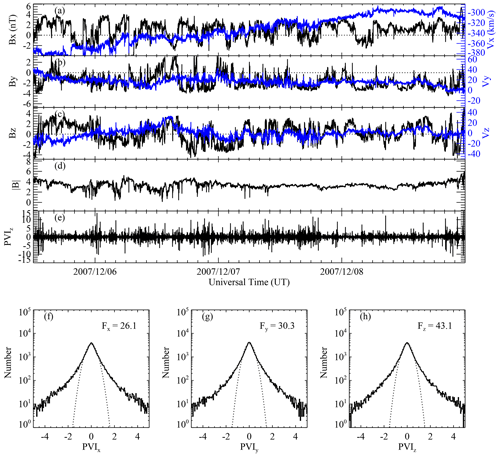Figure 1A typical slow-wind stream observed from 12:00:00 UT on 5 December 2007 to 00:00:00 UT on 9 December 2007 by the Wind spacecraft at the L1 point. (a–c) Time variations in the three components of the magnetic field vector (black) and proton velocity vector (blue) in the GSE coordinates. Horizontal dotted lines correspond to 0 nT. (d) Magnetic field magnitude. (e) Normalized partial variance of increments (PVI) for the z component of the magnetic field vector at the timescale of τ=24 s. (f–h) Probability distribution function (PDF, solid black curves) of the PVI for the three respective components of magnetic field. The flatness Fi ($i=x,y,z$) of each distribution is marked in each panel. Dotted curves denote the standard Gaussian distribution.

A parameter named normalized partial variance of increments (PVI) is applied to quantitatively analyze the intermittency in the solar wind turbulence, following previous studies . For a component of the magnetic field vector in a given slow-wind stream, the time series of the PVI is presented as follows:

$\begin{array}{}\text{(1)}& {\mathrm{PVI}}_{i}\left(t,\mathit{\tau }\right)=\frac{\mathit{\delta }{B}_{i}\left(t,\mathit{\tau }\right)}{\sqrt{<{\left|\mathit{\delta }{B}_{i}\left(t,\mathit{\tau }\right)\right|}^{\mathrm{2}}>}},\end{array}$

where Bi(t) is the time series of the i component of the magnetic field vector ($i=x,y,z$), $\mathit{\delta }{B}_{i}\left(t\right)={B}_{i}\left(t+\mathit{\tau }\right)-{B}_{i}\left(t\right)$, and $<\mathrm{\dots }>$ denotes an ensemble average in the given stream. The time lag τ is selected as 24 s following , corresponding to a spatial separation within the inertial range. In the following, we will refer to the PVIi(t,τ) as PVIi(t) for simplicity without further specification. Figure 1e shows the time series of PVIz(t) for the stream. Many spikes appear in the time series of PVIz(t), which correspond to large-amplitude fluctuations embedded in the background turbulence.

In Fig. 1f, g, and h, we demonstrate the probability distribution function (PDF, solid black curves) of the PVI for the three respective components of the magnetic field. The dotted curves are a standard Gaussian distribution, and they are plotted for easy comparison. The Gaussian distributions are located between the PVI range [$-\mathrm{2},\mathrm{2}$]. Beyond this range, the observed distribution curves exhibit long tails, and the tails extend even beyond the plotted range [$-\mathrm{5},\mathrm{5}$]. The PVI range [$-\mathrm{2},\mathrm{2}$] will then be used to select intermittent structures. Actually PVIz can achieve ±10, as shown in Fig. 1e. Thus, it is clear that the profiles of the PDF for the three components (PVIx, PVIy, and PVIz) all deviate significantly from the Gaussian distribution and have long tails when the absolute value of the PVIi increases. The long tails of the non-Gaussian PDF profiles indicate the existence of intermittent structures.

We calculate the flatness for each distribution as follows: ${F}_{i}=<\left({\mathrm{PVI}}_{i}\left(t\right){\right)}^{\mathrm{4}}>/<\left({\mathrm{PVI}}_{i}\left(t\right){\right)}^{\mathrm{2}}{>}^{\mathrm{2}}$, where $<\mathrm{\dots }>$ still denotes the ensemble average in the given stream. An empirical rule is that the minimum number N of data points in the time series to be used to accurately calculate moments of order M is $N={\mathrm{10}}^{M+\mathrm{1}}$ (see, e.g., ). In the case shown in Fig. 1, the total number of samples is 100 800. As flatness (the fourth-order moment) is considered here, the total number of samples meets the requirement, which is larger than the minimum number N=105. The flatness values are marked as Fx=26.1, Fy=30.3, and Fz=43.1 in Fig. 1f, g, and h, respectively. They are much larger than 3 (characteristic of a standard Gaussian distribution). This again indicates that the fluctuations are highly intermittent.

The criterion $|{\mathit{PVI}}_{i}\left(t\right)|>\mathrm{2}$ is applied for the basic identification of intermittent structures. First, we find the time instants that satisfy at least one of the following conditions: $|{\mathrm{PVI}}_{x}\left(t\right)|>\mathrm{2}$, $|{\mathrm{PVI}}_{y}\left(t\right)|>\mathrm{2}$, or $|{\mathrm{PVI}}_{z}\left(t\right)|>\mathrm{2}$. Some of the instants are isolated, whereas some of them are clustered and continuous. Structures are only chosen for the following study if the number of continuous instants is not smaller than 3. The remaining instants are ignored. A continuous series of intermittent instants is considered to be an intermittent structure. Moreover, if the number of instants between two adjacent structures is smaller than 3, the two structures and the data points between them are merged together and are seen as one “long-lived” structure. For a given structure, we use tB and tE to denote its beginning time and ending time, respectively, and we use $\left({t}_{\mathrm{E}}-{t}_{\mathrm{B}}\right)/\mathrm{\Delta }t$ as the width of the structure in the unit of data points. An interval between [tB−150 s, tE+150 s] is called an intermittent interval. After the 10 % data gap constraint is applied, we find 56 398 intermittent intervals from 46 slow-wind streams between 2005 and 2013.

Figure 2 shows a typical case of an intermittent interval observed by the Wind spacecraft on 23 February 2007. Figure 2a, b, and c present the time variations in the three components of the magnetic field vector (black) and the proton velocity vector (gray). The magnetic field data are transformed into Alfvén units (i.e., $B/\sqrt{{\mathit{\mu }}_{\mathrm{0}}{m}_{\mathrm{p}}〈{n}_{\mathrm{p}}〉}$, where μ0 is susceptibility, mp is proton mass, and np is the average proton number density of the  5 min interval), so that the fluctuation amplitudes of the magnetic field and the velocity are comparable. Figure 2d shows the time variations in the magnetic field magnitude. Figure 2e shows the time series of the PVIx(t) (purple), PVIy(t) (yellow), and PVIz(t) (green) as well as the trace PVI ($I=\sqrt{\left({\mathrm{PVI}}_{x}{\right)}^{\mathrm{2}}+\left({\mathrm{PVI}}_{y}{\right)}^{\mathrm{2}}+\left({\mathrm{PVI}}_{z}{\right)}^{\mathrm{2}}}$) (black). The two vertical dotted lines mark the beginning time (tB=01:44:19 UT) and ending time (tE=01:44:34 UT) of the intermittent structure, respectively. We see that $|{\mathrm{PVI}}_{z}|$ remains larger than 2 for 15 s (five data points) between the two vertical lines, which satisfies our criteria for intermittent structure selection. Accordingly, the width of this intermittent structure obtained from tEtB, during which the condition $|{\mathrm{PVI}}_{z}|>\mathrm{2}$ is satisfied, is recorded as 15 s (five data points).

In the case shown in Fig. 2, a very significant jump happens in the z component of the magnetic field between tB and tE. We also notice that, in this case, the fluctuation amplitude of the proton velocity is much smaller than the magnetic field (normalized residual energy of ${\mathit{\sigma }}_{\mathrm{r}}=-\mathrm{0.91}$), and the fluctuations between the velocity and the magnetic field are not well correlated (correlation coefficient of $\mathrm{cc}=-\mathrm{0.11}$). These characteristics indicate that this case may be associated with magnetic field directional turning . It is convected by the solar wind and has almost no velocity fluctuations. Hence, it can lead to a low normalized residual energy (close to −1) and a low correlation between B and V in the observations.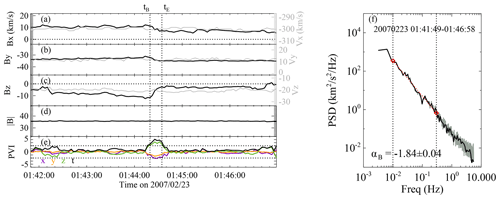Figure 2A typical case of an intermittent interval observed by the Wind spacecraft between 01:41:49 and 01:46:58 UT on 23 February 2007. (a–c) Time variations in the magnetic field vector (black) and the proton velocity vector (gray) in the GSE coordinates. The magnetic field is plotted in Alfvén units (i.e., $B/\sqrt{{\mathit{\mu }}_{\mathrm{0}}{m}_{\mathrm{p}}〈{n}_{\mathrm{p}}〉}$, where μ0 is susceptibility, mp is proton mass, and np is the average proton number density of this interval). (d) Magnetic field magnitude plotted in Alfvén units. (e) Normalized partial variance of increments (PVI) for the magnetic field vector at the timescale of τ=24 s, with PVIx in purple, PVIy in orange, PVIz in green, and the matrix trace of the PVI in black. The two horizontal lines correspond to $|\mathrm{PVI}|=\mathrm{2}$, which was used to search for the intermittent structure. The two vertical dotted lines mark the beginning time (tB) and the ending time (tE) of the intermittent structure, respectively. (f) Spacecraft-frame trace power spectra of magnetic field fluctuations. The gray curve corresponds to the magnetic power spectrum obtained by performing FFT on the $\mathrm{1}/\mathrm{11}$ s resolution magnetic field data in Alfvén units, still using $\mathbf{B}/\sqrt{{\mathit{\mu }}_{\mathrm{0}}{m}_{\mathrm{p}}〈{n}_{\mathrm{p}}〉}$ with a simple rectangular window. The black curve superposed on the gray one corresponds to the uniformly distributed spectrum after interpolation. The magnetic spectral index and its uncertainty are obtained by applying a least-squares fit to the interpolated spectrum, resulting in the straight line (dotted red line) over the frequency range from 0.01 to 0.3 Hz (between the two vertical dotted lines).

Next, we determine the intermittency level for each interval. The trace of the normalized partial variance of increments is obtained from $I=\sqrt{\left({\mathrm{PVI}}_{x}{\right)}^{\mathrm{2}}+\left({\mathrm{PVI}}_{y}{\right)}^{\mathrm{2}}+\left({\mathrm{PVI}}_{z}{\right)}^{\mathrm{2}}}$. If the maximum I (Imax) during an intermittent structure (e.g., between the two vertical lines for the case shown in Fig. 2) is also the maximum I during the corresponding intermittent interval (e.g., the whole interval for the case shown in Fig. 2), this interval will be reserved, and Imax is recorded as the intermittency level of this case. Otherwise, the case is eliminated because the energy of the fluctuations during the interval is not dominated by the intermittent structure of interest. In the case shown in Fig. 2, we see that Imax=4.10 at 01:44:23 UT is also the maximum I within the plotted interval, so this case satisfies the condition well. In this way, 25 912 intermittent intervals are reserved for the following analysis.

We then perform fast Fourier transform (FFT) on the magnetic field fluctuations in Alfvén units and obtain the spectral index of the Fourier spectrum in the inertial range. In this procedure, we use high-resolution magnetic field data with $\mathrm{\Delta }{t}_{\mathrm{H}}=\mathrm{1}/\mathrm{11}$ s, so that the magnetic spectral index will be more reliable. The high-resolution magnetic field data are first transformed into Alfvén units (i.e., $B/\sqrt{{\mathit{\mu }}_{\mathrm{0}}{m}_{\mathrm{p}}〈{n}_{\mathrm{p}}〉}$, where np is the average proton number density of each interval). When converting the magnetic field into Alfvén units, we use one proton number density value that corresponds to the ensemble average of the proton number density np for each selected interval. By doing so, we avoid contamination of the magnetic spectral index value by noise in the density measurements, which would result from using a density value that changes every 3 s. For a given intermittent interval, the time series of each component of the high-resolution magnetic field data in Alfvén units is Fourier transformed using the FFT method with a simple rectangular window. This method could introduce extra discontinuity in the data that will add Fourier power to the magnetic power spectral density (PSD), as mentioned by and . In Sect. 4.4, we linearly detrend the data prior to Fourier transformation, following , and make a comparison between the two methods. The trace of the magnetic spectral matrix gives the total power spectral density, and the magnetic spectrum is then smoothed using the three-point centered moving average following . In Fig. 2f, we plot the magnetic PSD as a function of the spacecraft frequency (f) in log–log space, i.e., y=log 10(PSD) versus x=log 10(f), shown using the gray curve. We see that, using the high-resolution data, the magnetic spectrum can cover more than 3 orders of magnitude from $\mathrm{3.3}×{\mathrm{10}}^{-\mathrm{3}}$ to 5.5 Hz.

It is known that the points of the gray spectrum shown in Fig. 2f are not uniformly distributed in the logarithmic space of f. As mentioned in , the number of data points between two points (x and xx) in this space increases exponentially with x. If a least-squares fit is performed, each point has equal weight. Therefore, the fit favors the points in the higher-frequency range, as this range contains more points . In order to avoid this issue, we linearly interpolate the spectral density onto a uniformly spaced grid with $\mathrm{\Delta }x=\left({f}_{\mathrm{max}}-{f}_{\mathrm{min}}\right)/\mathrm{100}$ in the log–log space, following . In Fig. 2f, the black curve superposed on the original gray spectrum demonstrates the interpolated spectrum.

We then perform a least-squares fit to the interpolated magnetic spectrum to obtain the magnetic spectral index αB in the log–log space in the inertial range. The least-squares fit is performed in the frequency range between 0.01 and 0.3 Hz (between the two vertical dotted lines shown in Fig. 2f), and the magnetic spectrum can be fitted well by a straight line with a slope of αB in this range. The slope (αB) and its corresponding error (${\mathrm{\Delta }}_{{\mathit{\alpha }}_{B}}$) are obtained (and both marked in Fig. 2f) as ${\mathit{\alpha }}_{B}=-\mathrm{1.84}±\mathrm{0.04}$. We perform the same analysis on all of the selected intervals. The cases with a relative fitting error ${\mathrm{\Delta }}_{{\mathit{\alpha }}_{B}}/{\mathit{\alpha }}_{B}>\mathrm{5}\phantom{\rule{0.125em}{0ex}}\mathit{%}$ are eliminated, as their magnetic spectra do not have a good power-law shape and cannot be fitted well by a straight line in the log–log space at the frequency range of interest. Finally, 24 886 intermittent intervals are reserved for the following statistical analysis in order to explore the relation between the magnetic spectral index αB and the intermittency level Imax.

3 Results: variations in the magnetic spectral index versus the intermittency level

For the 24 886 selected cases, we first present the joint distribution of their width in units of data points and intermittency level Imax in Fig. 3a. Here, the width in units of data points for an intermittent structure is obtained from tEtB, during which the condition $|{\mathrm{PVI}}_{i}|>\mathrm{2}$ satisfies (i=x, y, or z), divided by the time resolution Δt=3 s. We see that most of the cases have the following values: $\mathrm{5}\le \mathrm{width}<\mathrm{7}$ and $\mathrm{3}\le {I}_{\mathrm{max}}<\mathrm{6}$. As the width increases, the distribution of Imax extends to a wider range. This phenomenon makes the pattern of the joint distribution look like a triangle, which is consistent with . In their Fig. 8, the aforementioned authors show the triangle-like shape of the 2D distribution in the Δθτ plane, where Δθ and τ are the deflection angle across the current sheet and the width of the current sheet, respectively. Figure 3b shows the probability distribution of the width for the intermittent structures of interest. The width extends from 3 (9 s) to 20 data points (60 s), and the most probable value is 5 data points (15 s). As the width increases, the probability distribution function first increases immediately and then decreases gradually. Figure 3c shows the probability distribution of the intermittency level Imax. The value of Imax extends from about 2 to 15, and the most probable value is 4.5. The profile of the distribution is similar to that of the width.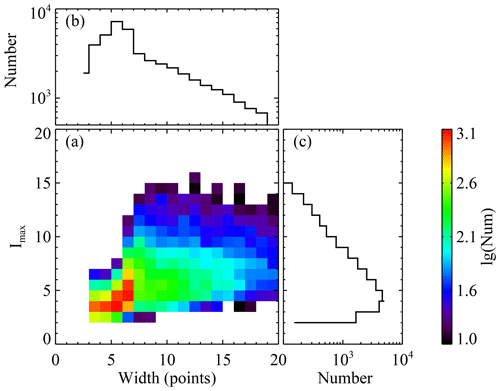Figure 3(a) Joint distribution of width in units of data points and intermittency level (Imax) for the 24 886 selected intermittent structures. The width in units of data points for an intermittent structure is obtained from tEtB, during which the condition $|{\mathrm{PVI}}_{i}|>\mathrm{2}$ satisfies (i=x, y, or z), divided by the time resolution Δt=3 s. The pixels containing no more than 10 cases are ignored. Panels (b) and (c) show the probability distribution of the width and of the intermittency level (Imax), respectively.

Another typical intermittent interval observed on 12 September 2010 is shown in Fig. 4, although with a higher intermittency level Imax=13.09. Figure 4 is plotted in the same format as Fig. 2. The intermittent structure is marked by the two vertical dotted lines. Between the two vertical lines, the time instants all satisfy at least one of the abovementioned conditions: $|{\mathrm{PVI}}_{x}|>\mathrm{2}$, $|{\mathrm{PVI}}_{y}|>\mathrm{2}$, or $|{\mathrm{PVI}}_{z}|>\mathrm{2}$. Between tB and tE, a large jump happens in both the x and z components of the magnetic field. The fluctuations in the proton velocity (in gray) are well correlated with the fluctuations in the magnetic field (correlation coefficient of cc=0.97). However, the fluctuation amplitude of the proton velocity is much smaller than the magnetic field (normalized residual energy of ${\mathit{\sigma }}_{\mathrm{r}}=-\mathrm{0.50}$). This indicates that this may be a magnetic–velocity alignment structure . Magnetic–velocity alignment structures, the generation mechanism of which remains unclear, are a kind of magnetically dominated structure but with a high correlation between the magnetic field fluctuations and velocity fluctuations. For these kinds of structures, the magnetic field fluctuations are nearly aligned with the velocity fluctuations.

For the case shown in Fig. 4, the intermittency level Imax is recorded as 13.09, which corresponds to the value of I at 06:28:48 UT. Figure 4f shows the power spectrum of magnetic field fluctuation obtained by performing FFT on the high-resolution magnetic field data. The original spectrum before interpolation is still plotted in gray, and the uniformly distributed spectrum after interpolation in black is superposed on the gray one. A least-squares fit is performed on the interpolated spectrum at the frequency range between 0.01 and 0.3 Hz. The spectral index is obtained as ${\mathit{\alpha }}_{B}=-\mathrm{2.01}±\mathrm{0.04}$. The small fitting error indicates that the magnetic spectrum has a good power-law shape. The magnetic spectral index obtained here is very close to −2. Thus, it is very consistent with previous theory and observations, which proposed that the discontinuities can produce an f−2 energy spectrum in the inertial range .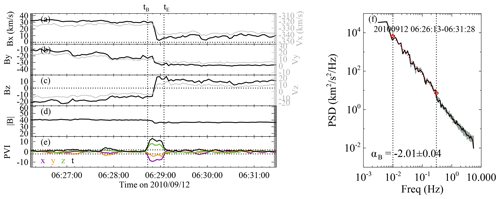Figure 4A typical case of an intermittent interval observed by the Wind spacecraft between 06:26:13 and 06:31:28 UT on 12 September 2010, displayed in the same format as Fig. 2.

However, as seen from the case with ${\mathit{\alpha }}_{B}=-\mathrm{1.84}±\mathrm{0.04}$, shown in Fig. 2, the discontinuities are not always related to a −2 magnetic spectral index in the solar wind observations. The case shown in Fig. 2 also has a typical discontinuity embedded in the background turbulence, but its intermittency level (Imax=4.10) is relatively smaller than that shown in Fig. 4 (Imax=13.09). Correspondingly, the magnetic spectrum of the case shown in Fig. 2 is shallower. Therefore, it is clear that the intermittency level can significantly affect the spectral index of the magnetic field fluctuations in the inertial range. It is necessary to know the analytical relation between the intermittency level and the magnetic spectral index.

In order to give the continuous variation in the magnetic spectral index as a function of the intermittency level, we also select some “quiet” intervals with $|{\mathrm{PVI}}_{i}|<\mathrm{2}$. In this procedure, we first cut the data in the 46 slow-wind streams into short intervals with a duration of 5 min. We then check the maximums of $|{\mathrm{PVI}}_{x}|$, $|{\mathrm{PVI}}_{y}|$, and $|{\mathrm{PVI}}_{z}|$ in each interval, respectively. If their maximums are all smaller than 2, the interval is reserved as a quiet interval. During a given interval, the maximum of the trace $I=\sqrt{\left({\mathrm{PVI}}_{x}{\right)}^{\mathrm{2}}+\left({\mathrm{PVI}}_{y}{\right)}^{\mathrm{2}}+\left({\mathrm{PVI}}_{z}{\right)}^{\mathrm{2}}}$ is recorded as the “intermittency level” (Imax), although it may not be intermittent at all. Their magnetic spectral indices are also obtained using the method mentioned above. Subsequently, we find 17 386 quiet cases for the following study.

Figure 5 shows (using the same format as Fig. 2) a typical quiet interval with very low intermittency level of Imax=1.44. The magnetic power spectrum is much shallower than that of the intermittent intervals with relatively higher intermittency levels shown in Figs. 2 and 4. The magnetic spectral index comes out to be $-\mathrm{1.65}±\mathrm{0.04}$. It seems to be close to the Kolmogorov scaling of ${f}^{-\mathrm{5}/\mathrm{3}}$. We check the Alfvénicity of this case and find that it is not an Alfvénic interval with a low normalized cross helicity of σc=0.34 and a low Alfvén ratio of γA=0.47. It is worth noting that an intermittency correction could be considered if the magnetic spectrum scales as ${f}^{-\mathrm{5}/\mathrm{3}}$ for an Alfvénic interval.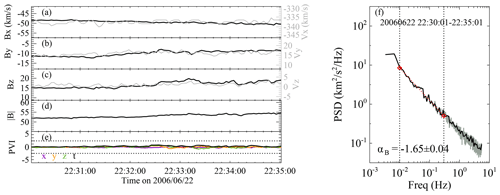Figure 5A typical case of a quiet interval observed by the Wind spacecraft between 22:30:01 and 22:35:01 UT on 22 June 2006, shown in the same format as Fig. 2. The fluctuation amplitude of the proton velocity (gray curves in panels a, b, and c) is very close to the instrument noise level (2 km s−1) in the 3DP velocity observations . Thus, the variations in the proton velocity look noisy here.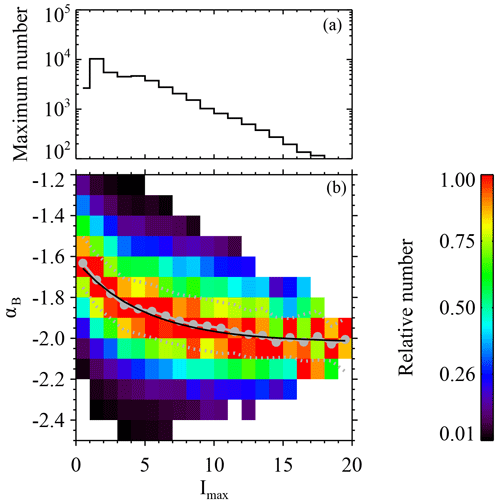Figure 6Joint distribution of the intermittency level Imax and magnetic spectral index αB for the 42 272 selected intervals. The bin width of Imax is 1, and the bin width of αB is 0.1. For a given pixel, the color denotes the relative number, which is the number of cases normalized by the maximum number in the corresponding column (Imax bin). The maximum number in each bin is shown in panel (a). The pixels containing no more than 10 cases are ignored. The solid gray circles represent the average αB in each Imax bin. The dotted gray lines represent the upper/lower quartiles. The black curve, corresponding to the exponential function ${\mathit{\alpha }}_{B}=\mathrm{0.4}\mathrm{exp}\left(-{I}_{\mathrm{max}}/\mathrm{5}\right)-\mathrm{2.02}$, represents the fitting result to the solid gray circles.

Figure 6b shows the joint distribution of Imax and αB for the 42 272 selected intervals. The x axis, corresponding to the intermittency level Imax in the range [0,20], is divided into 20 bins. The y axis, corresponding to the magnetic spectral index αB in the range $\left[-\mathrm{2.5},-\mathrm{1.2}\right]$, is divided into 13 bins. For a given pixel, the color denotes the number of cases normalized by the maximum number of pixels in the corresponding Imax bin. Thus, in each column, the pixel with the largest number of cases is colored in red, corresponding to 1. The maximum number in each column versus Imax is also shown in Fig. 6a. In order to guarantee that there are enough cases used for the statistics, the pixels containing no more than 10 cases are ignored. Therefore, the pixels in black contain the smallest number of cases, but the number of cases is still larger than 10. If we focus on the pixels in red, we notice that the magnetic spectral index αB has a very clear decreasing trend from $\sim -\mathrm{1.6}$ to $\sim -\mathrm{2}$ as the intermittency level Imax increases. The solid gray circles show the average αB in each Imax bin as a function of Imax, and the dotted gray lines represent the upper/lower quartiles. It is found that the magnetic power spectrum gets steeper quickly from ${f}^{-{\mathrm{1.63}}_{-\mathrm{0.12}}^{+\mathrm{0.09}}}$ to ${f}^{-{\mathrm{1.84}}_{-\mathrm{0.11}}^{+\mathrm{0.14}}}$ as Imax increases from 0.5 to 3.5. When Imax increases from 4.5 to 15.5, the magnetic power spectrum gets steeper slowly from ${f}^{-{\mathrm{1.86}}_{-\mathrm{0.11}}^{+\mathrm{0.14}}}$ to ${f}^{-{\mathrm{1.99}}_{-\mathrm{0.11}}^{+\mathrm{0.09}}}$. As Imax>16, the magnetic spectral index remains close to −2.

The observed variation in the magnetic spectral index αB versus the intermittency level Imax can be well fitted by an exponential function. In Fig. 6, the black curve corresponding to ${\mathit{\alpha }}_{B}=\mathrm{0.4}\mathrm{exp}\left(-{I}_{\mathrm{max}}/\mathrm{5}\right)-\mathrm{2.02}$ shows the fitting result. This empirical relation supplies the continuous variation in the spectral index αB of the magnetic field in the inertial range as a function of the intermittency level Imax. The empirical relation tells us that the magnetic spectral index in the inertial range will be close to −1.6 when Imax is small (and the fluctuations in the magnetic field could be considered to be randomly distributed). As the fluctuations become intermittent, the magnetic spectrum gradually becomes steeper until $\sim {f}^{-\mathrm{2}}$.

4 Discussion

Our result confirms the idea that intermittent structures have a significant influence on the magnetic spectral index and often make the spectra become steeper . It is generally acknowledged that the time series of discontinuities produce an f−2 energy spectrum in the inertial range. More recently, researchers have found that discontinuities can also produce shallower magnetic spectra . In previous studies, discontinuities in the solar wind have been identified mainly as rotational discontinuities . However, in some other studies, discontinuities have been identified mainly as tangential discontinuities, depending on the different techniques used for data analysis . Here, from the continuous relation, we find that f−2 scaling could be produced if the intermittency level of the structure embedded in the turbulence was high enough, i.e., Imax>15 for the cases studied in this work. We have examined whether the intermittency level Imax could be biased by the anisotropy of fluctuations. It was found that the intermittency level Imax appears not to be dependent on the direction of the predominant fluctuations (figure not shown here, as it is similar to Fig. 9 shown below).

Our result is also consistent with the radial evolution trend in intermittency and the magnetic spectral index in the solar wind. The evolution of intermittency with distance from the Sun can be explained on the basis of the interplay between coherent (intermittent) structures and Alfvénic fluctuations. Intermittent events advected by the wind are increasingly exposed as Alfvénic fluctuations are depleted with the heliocentric distance (see, e.g., ). Using the observations from the Parker Solar Probe, researchers have also found that there is a clear transition for the magnetic spectral index in the inertial range as the radial distance from the Sun increases . When r≈0.17 AU, the magnetic spectral index is close to $-\mathrm{3}/\mathrm{2}$. When r≈0.6 AU, the magnetic spectrum becomes steeper as $\mathit{\alpha }\approx -\mathrm{5}/\mathrm{3}$. These observational results indicate that the solar wind turbulence becomes more intermittent as r increases and that the magnetic spectrum gets steeper. The variation trend in the magnetic spectral index versus the intermittency is confirmed by our observations. Recently, several papers have been published on scaling properties and intermittency levels with respect to the Parker Solar Probe .

We also notice that the magnetic spectral index of the intervals for the cases with a very low intermittency level $\mathrm{0}<{I}_{\mathrm{max}}<\mathrm{1}$ is between −1.62 and −1.69, which is close to the Kolmogorov scaling. This is different from , who found that current-sheet-free periods show ${f}^{-\mathrm{3}/\mathrm{2}}$ Iroshnikov–Kraichnan scaling. From Fig. 6, we see that some of the low-intermittency-level cases can also produce ${f}^{-\mathrm{3}/\mathrm{2}}$ scaling, but the cases with $-\mathrm{1.6}<{\mathit{\alpha }}_{B}<-\mathrm{1.4}$ only account for 20 % of all of the cases with Imax<1. The differences between this work and include the following: (1) the aforementioned authors focus on the  1 d Ulysses data at about 5 AU, whereas we use the 5 min Wind data at about 1 AU; (2) the frequency range for the fit is [${\mathrm{10}}^{-\mathrm{3}},{\mathrm{10}}^{-\mathrm{1}}$] Hz in , whereas it is [0.01,0.3] Hz here.

f−2 scaling has been reported for parallel-sampling magnetic fluctuations in many previous studies associated with magnetic spectral anisotropy . found that the magnetic spectrum in the parallel direction becomes shallower from f−2 to ${f}^{-\mathrm{5}/\mathrm{3}}$ after removing the intermittency. However, the question regarding how intermittency affects the anisotropy of the magnetic spectral index remains unclear. In the future, we might try to check the intermittency level of the parallel-sampling data to see if the steep spectrum in the parallel direction is related to a high intermittency level or not.

Intermittency in many theoretical models has also been found to steepen the inertial-range power spectrum of turbulence. For example, a multifractal model developed by (SL model) provided intermittency correction to the Kolmogorov law and predicted an energy spectrum of $E\left(k\right)\approx {k}^{-\mathrm{5}/\mathrm{3}-\mathrm{0.03}}$ for fluids. presented a magnetohydrodynamic (MHD) cascade model and provided an intermittency modification to the Kraichnan theory. extended the SL model to the MHD case, and the energy spectrum was obtained as $E\left(k\right)\approx {k}^{-\mathrm{3}/\mathrm{2}-\mathrm{0.04}}$. For the velocity spectrum,  predicted $E\left(k\right)\approx {k}^{-\mathrm{1.74}}$ from an analytical study of driven supersonic MHD turbulence. Recently, found that the power spectrum of the intermittent turbulence flattens when considering scale-dependent dynamic alignment. However, there seems to be no conclusion regarding which model is the most appropriate one to describe the solar wind turbulence. According to the observational result shown in this work with respect to the slow-wind streams, we obtain an empirical relation between the magnetic spectral index αB and the intermittency level Imax. This relation will supply an observational basis for theoretical studies of the intermittent turbulence and will help improve the turbulence theory related to the slow solar wind.

## 4.1 Influence of magnetic compressibility

Besides intermittency, the magnetic spectral index has been reported to also depend on the level of magnetic compressibility. Magnetic compressibility has been defined as the ratio between the variance in the magnetic field magnitude fluctuations and the variance matrix trace of the fluctuations, i.e., ${c}_{b}={\mathit{\sigma }}_{|B|}^{\mathrm{2}}\phantom{\rule{0.25em}{0ex}}/\phantom{\rule{0.25em}{0ex}}{\sum }_{i=x,y,z}{\mathit{\sigma }}_{{B}_{i}}^{\mathrm{2}}$ . Here, in order to take the influence of the magnetic compressibility on the shape of the magnetic spectrum into account, we also calculate the magnetic compressibility of all of the 24 886 intermittent intervals and the 17 386 quiet intervals in the 46 slow-wind streams.

Figure 7b shows (in the same format as Fig. 6) the joint distribution of the magnetic compressibility cb and αB for the 24 886 selected intermittent intervals. The x axis, corresponding to the magnetic compressibility cb in the range [0,0.5], is divided into 20 bins. The y axis, corresponding to the magnetic spectral index αB in the range $\left[-\mathrm{2.5},-\mathrm{1.2}\right]$, is still divided into 13 bins. For a given pixel, the color also denotes the number of cases normalized by the maximum number of pixels in the corresponding cb bin. The maximum number in each column versus cb is also shown in Fig. 7a. The pixels containing no more than 10 cases are ignored. When we focus on the pixels in red, we notice that the magnetic spectral index αB remains almost constant as cb increases. The solid gray circles show the average αB in each cb bin, and the two dotted gray lines represent the respective upper and lower quartiles. For the selected intermittent intervals, it is found that the average slope of the magnetic spectrum in the inertial range varies between $\left[-\mathrm{1.90},-\mathrm{1.80}\right]$ as the magnetic compressibility cb increases from 0 to 0.5 and that there is no systematic trend. This result could indicate that, for the intermittent cases, the magnetic compressibility does not have significant influence on the magnetic spectral index in the slow-wind streams of interest.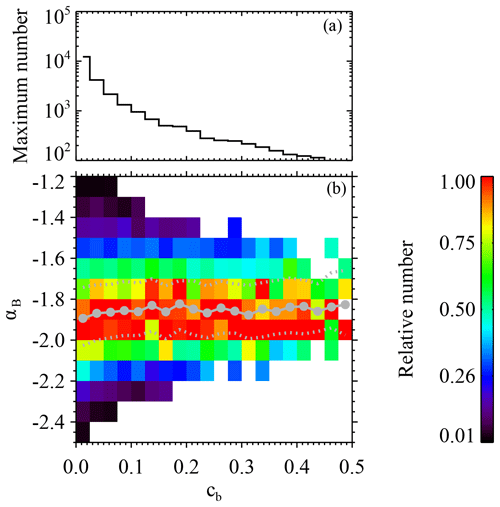Figure 7Joint distribution of the magnetic compressibility cb and magnetic spectral index αB for the 24 886 selected intermittent intervals, shown in the same format as Fig. 6.

The same analysis is performed on the 17 386 selected quiet intervals. The result is shown in Fig. 8. Figure 8 is plotted in the same format as Fig. 7. When we focus on the most probable value of αB in each cb bin, i.e., the pixels in red, we also find that no clear trend appears. The solid gray circles and the two dotted gray lines represent the average αB in each cb bin and the upper/lower quartiles, respectively. When cb increases from 0 to 0.5, the magnetic spectral index changes slightly from $-\mathrm{1.76}±\mathrm{0.14}$ to $-\mathrm{1.70}±\mathrm{0.10}$. This result indicates that, for the quiet cases in the slow-wind streams of interest, the magnetic compressibility also does not significantly affect the magnetic spectral index.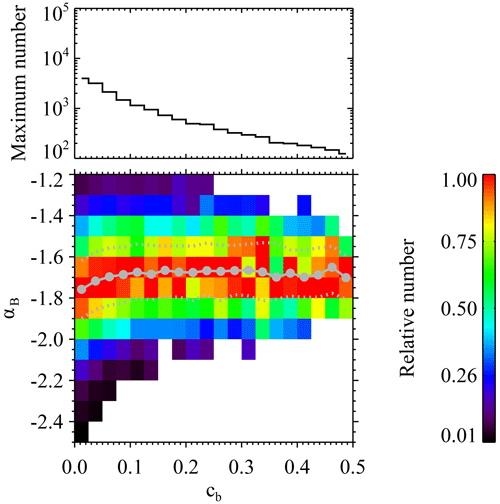Figure 8Joint distribution of the magnetic compressibility cb and magnetic spectral index αB for the 17 386 selected quiet intervals, shown in the same format as Fig. 6.

## 4.2 Influence of anisotropy of magnetic field fluctuations

As different spectral indices are observed in different directions with respect to the mean field, as mentioned in Sect. 1, it is necessary to reveal how the presented results shown in Fig. 6 could be biased by the anisotropy of magnetic field fluctuations. Thus, we perform a check to see if the spectral slope is dependent on the predominance of fluctuations in a specific direction. Here, the direction of the predominant fluctuations is indicated by the maximum variance (L) direction, which is obtained via minimum variance analysis . In Fig. 9, we show the variations in the magnetic spectral index as a function of the angle between the L and i direction (θLi) (where i denotes the x axis, y axis, and z axis of GSE coordinates) as well as the variations in the spectral index versus the angle between L and the mean magnetic field direction of each interval (θLB).

Figure 9a2 shows the variation in the magnetic spectral index αB as a function of θLX. The angle ${\mathit{\theta }}_{LX}\sim \mathrm{0}{}^{\circ }$ means that the predominant fluctuations in the intermittent structure are mainly focused in the x direction, whereas ${\mathit{\theta }}_{LX}\sim \mathrm{90}{}^{\circ }$ means that they are mainly focused in the plane perpendicular to the x direction. Only 79 % of the selected intervals with ${\mathit{\lambda }}_{\mathrm{1}}/{\mathit{\lambda }}_{\mathrm{2}}>\mathrm{3}$ remain for the analysis, where λ1 and λ2 are the eigenvalues corresponding to the maximum variance direction and the intermediate variance direction, respectively. This condition guarantees that the maximum variation direction is determined precisely, and the fluctuations in the L direction are distinctly dominant in each interval. Figure 9a1 and a2 are plotted in a similar format to Fig. 6. For a given pixel in Fig. 9a2, the color denotes the number of cases normalized by the maximum number in the corresponding θLX bin, and the maximum number in each bin is shown in Fig. 9a1. The solid gray circles represent the average αB in each θLX bin. The dotted gray lines represent the upper/lower quartiles. The solid gray circles show that there is a slight decreasing trend for the average spectral index αB (from −1.76 to −1.86) as θLX increase from 0 to 90. However, if we consider the quartiles (i.e., from $-{\mathrm{1.76}}_{-\mathrm{0.10}}^{+\mathrm{0.14}}$ to $-{\mathrm{1.86}}_{-\mathrm{0.14}}^{+\mathrm{0.13}}$), the slight trend is nearly negligible. Therefore, the magnetic spectral indices of the intervals with the predominant fluctuations parallel or perpendicular to the x direction are not significantly different.

Figure 9b, c, and d show the variation in the magnetic spectral index as a function of θLY, θLZ, and θLB, respectively. A slight increasing trend (from $-{\mathrm{1.89}}_{-\mathrm{0.17}}^{+\mathrm{0.17}}$ to $-{\mathrm{1.84}}_{-\mathrm{0.13}}^{+\mathrm{0.14}}$) appears in Fig. 9b2, but the trend is also not significant, considering the errors. In Fig. 9c2, the average αB (solid gray circles) remains almost constant at −1.85. In Fig. 9d2, the average αB (solid gray circles) varies with θLB, and no clear trend exists.

According to the results presented in Fig. 9, we suggest that the influence of the anisotropy of the predominant fluctuations on the magnetic spectral index is not as significant as the influence of the intermittency level Imax on the index (when Imax increases from 0 to 20, αB decreases from −1.63 to −2.01).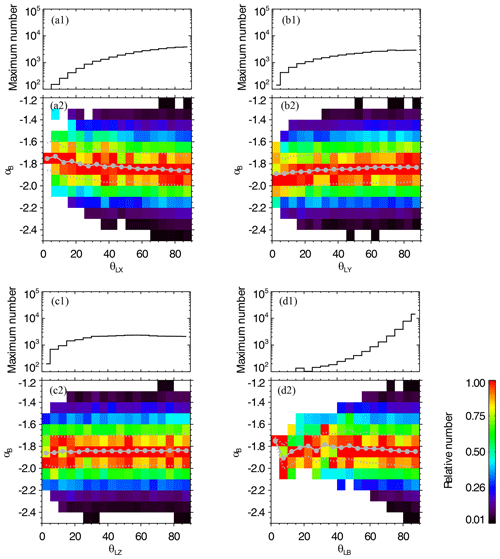Figure 9(a2) Joint distribution of θLX and the magnetic spectral index αB for the 33 261 intervals with ${\mathit{\lambda }}_{\mathrm{1}}/{\mathit{\lambda }}_{\mathrm{2}}>\mathrm{3}$. For a given pixel, the color denotes the relative number, which is the number of cases normalized by the maximum number in the corresponding θLX bin. The maximum number in each bin is shown in panel (a1). The pixels containing no more than 10 cases are ignored. The solid gray circles represent the average αB in each θLX bin. The dotted gray lines represent the upper/lower quartiles. Panels (b1) and (b2) are plotted in the same format as panels (a1) and (a2) but for θLY. Panels (c1) and (c2) correspond to θLZ. Panels (d1) and (d2) correspond to θLB.

## 4.3 Coincidence between the intermittency level and multifractal width

As shown in the literature , intermittency is strictly related to multifractality, which is measured by looking at the high-order scaling properties. Therefore, it is necessary to check if the Imax used here is consistent with multifractal indicators of intermittency, such as the multifractal width introduced in a series of work by Macek et al. (2005, 2009, 2012).

The multifractal properties can be described by the multifractal singularity spectrum of the observed time sequence. The width of the spectrum represents the extent of multifractality. Here, we estimate the multifractal singularity spectrum of the magnetic field fluctuations using the classical approach following previous studies . For each selected interval, we perform a multifractal analysis on the time sequence of the magnetic field fluctuations in the maximum variance direction (BL(t)) with a high time resolution of $\mathrm{\Delta }{t}_{\mathrm{H}}=\mathrm{1}/\mathrm{11}$ s. The increment of BL(t) is $\mathrm{\Delta }{B}_{L}\left(t\right)=|{B}_{L}\left(t+\mathrm{d}t\right)-{B}_{L}\left(t\right)|$, where dt=10 s belongs to the inertial range. The time series ΔBL(i) ($i=\mathrm{1},\mathrm{2},\mathrm{\dots },N$, where $N=T/\mathrm{\Delta }{t}_{\mathrm{H}}$ and T is the duration of each interval) is divided into subsets of variable scale Δ s, with $j=\mathrm{1},\mathrm{2},\mathrm{\dots },M$ ($M=T/\mathrm{\Delta }\phantom{\rule{0.125em}{0ex}}s$). A logarithmically spaced range of eight timescales $\mathrm{10}/\mathrm{11}$ s $<\mathrm{\Delta }\phantom{\rule{0.125em}{0ex}}s<\mathrm{150}$ s is used. For each subset, the generalized probability measure is defined as follows:

$\begin{array}{}\text{(2)}& {\mathit{\mu }}_{j}\left(\mathrm{\Delta }\phantom{\rule{0.125em}{0ex}}s\right)=\frac{{\sum }_{i=\left(j-\mathrm{1}\right)\mathrm{\Delta }\phantom{\rule{0.125em}{0ex}}s+\mathrm{1}}^{j\mathrm{\Delta }\phantom{\rule{0.125em}{0ex}}s}|\mathrm{\Delta }{B}_{L}\left(i\right)|}{{\sum }_{i=\mathrm{1},N}|\mathrm{\Delta }{B}_{L}\left(i\right)|}.\end{array}$

For a given q, we calculate the q-order total probability measure, and it scales as follows:

$\begin{array}{}\text{(3)}& {\mathit{\chi }}_{q}\left(\mathrm{\Delta }\phantom{\rule{0.125em}{0ex}}s\right)=\sum _{j=\mathrm{1}}^{M}\mathrm{\Delta }\phantom{\rule{0.125em}{0ex}}s|{\mathit{\mu }}_{j}\left(\mathrm{\Delta }\phantom{\rule{0.125em}{0ex}}s\right){|}^{q}\propto \left(\mathrm{\Delta }\phantom{\rule{0.125em}{0ex}}s{\right)}^{{\mathit{\tau }}_{q}},\end{array}$

where $q\in \left[-\mathrm{5},\mathrm{5}\right]$ with a step $\mathrm{d}q=\mathrm{1}/\mathrm{3}$ (similar to ). The scaling exponent τq is obtained by performing a linear fit of χq(Δ s) versus Δ s in the inertial range [8 s, 100 s] on a log–log plot. We then obtain the singularity spectrum from $f\left(\mathit{\alpha }\right)=q{\mathit{\alpha }}_{q}-{\mathit{\tau }}_{q}$ and ${\mathit{\alpha }}_{q}=\mathrm{d}{\mathit{\tau }}_{q}/\mathrm{d}q$ . Figure 10a presents the variations in f(α) versus α, with red denoting the intermittent interval shown in Fig. 4 (Imax=13.09), black denoting the intermittent interval shown in Fig. 2 (Imax=4.10), and blue denoting the quiet interval shown in Fig. 5 (Imax=1.44). The dots and solid lines represent the observational results and cubic polynomial fit to them, respectively.

A quantitative description of the degree of multifractality is the width of the singularity spectrum $\mathrm{\Delta }\mathit{\alpha }={\mathit{\alpha }}_{\mathrm{max}}-{\mathit{\alpha }}_{\mathrm{min}}$. We estimate αmin and αmax by fitting the observed values of (α,f(α)) with the cubic polynomial and extrapolating to f(α)=0, as shown in Fig. 10a. We find that the multifractal widths of the two intermittent intervals (Δα=1.19 in red and Δα=1.16 in black) are both much larger than that of the quiet interval (Δα=0.81 in blue). Moreover, the intermittent interval with a higher level of intermittency (Imax=13.09) shown in red also corresponds to a wider singularity spectrum (Δα=1.19) compared with the one shown in black (Imax=4.10 and Δα=1.16).

In Fig. 10b, we show the statistical results of the multifractal width Δα versus the level of intermittency Imax for the 33 261 intervals with ${\mathit{\lambda }}_{\mathrm{1}}/{\mathit{\lambda }}_{\mathrm{2}}>\mathrm{3}$, as mentioned in Sect. 4.2. They are found to be positively correlated. When Imax<3, the multifractal width Δα rapidly increases from 0.8 to 1.05. When Imax>3, Δα increases slowly from 1.05 to  1.2. Accordingly, we suggest that, to some extent, the multifractal width Δα and the level of intermittency Imax coincide with each other.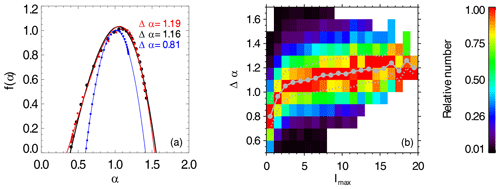Figure 10(a) Multifractal singularity spectra f(α) versus α observed in the slow wind (points), where red represents the intermittent interval shown in Fig. 4 (Imax=13.09), black represents the intermittent interval shown in Fig. 2 (Imax=4.10), and blue represents the quiet interval shown in Fig. 5 (Imax=1.44). The solid lines denote the cubic polynomial fit to the observations. The width of each singularity spectrum $\mathrm{\Delta }\mathit{\alpha }={\mathit{\alpha }}_{\mathrm{max}}-{\mathit{\alpha }}_{\mathrm{min}}$ is marked in the panel. (b) Joint distribution of Imax and Δα for the 33 261 intervals with ${\mathit{\lambda }}_{\mathrm{1}}/{\mathit{\lambda }}_{\mathrm{2}}>\mathrm{3}$. For a given pixel, the color denotes the relative number, which is the number of cases normalized by the maximum number in the corresponding Imax bin. The pixels containing no more than 10 cases are ignored. The solid gray circles represent average Δα in each Imax bin. The dotted gray lines represent the upper/lower quartiles.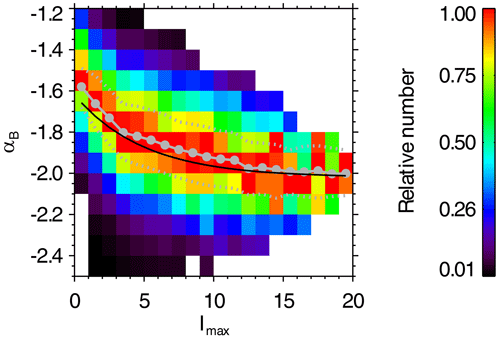Figure 11Joint distribution of the intermittency level Imax and magnetic spectral index αB obtained using the “linear detrending preparation” method, plotted in the same format as Fig. 6b. The black curve is the exponential function ${\mathit{\alpha }}_{B}=\mathrm{0.4}\mathrm{exp}\left(-{I}_{\mathrm{max}}/\mathrm{5}\right)-\mathrm{2.02}$ adopted from Fig. 6.

## 4.4 Linearly detrending data prior to FFT

When performing the FFT on the components of magnetic field data, we use a simple rectangular window (hereinafter referred to as the “no data preprocessing” method). This method could introduce extra discontinuity in the data that will add Fourier power to the magnetic PSD, as mentioned by and . Following , we try linearly detrending each data interval prior to Fourier transformation (hereinafter referred to as the “linear detrending preparation” method), and we compare the result with that in Fig. 6 obtained using the no data preprocessing method.

Figure 11 presents (plotted in the same format as Fig. 6b) the joint distribution of the intermittency level Imax and magnetic spectral index αB obtained using the linear detrending preparation method. The analytical relationship ${\mathit{\alpha }}_{B}=\mathrm{0.4}\mathrm{exp}\left(-{I}_{\mathrm{max}}/\mathrm{5}\right)-\mathrm{2.02}$, adopted from Fig. 6, is superposed on the figure as a black curve for easier comparison. It is clear that, when Imax>12, the black curve coincides with the averaged magnetic spectral indices αB (gray dots) well. However, when Imax<12, the averaged magnetic spectral indices αB (gray dots) obtained from the linear detrending preparation method appear to be larger than those obtained from the no data preprocessing method (denoted by the black curve). The differences between them are about 0.01–0.06. This is consistent with , who mentioned that the no data preprocessing method leads to slightly steeper spectral indices. When looking at the upper/lower quartiles, we notice that the distribution of αB in an Imax bin obtained using the linear detrending preparation method (e.g., ${\mathit{\alpha }}_{B}=-{\mathrm{1.90}}_{-\mathrm{0.14}}^{+\mathrm{0.15}}$ at Imax=8.5) is slightly wider than that obtained using the no data preprocessing method (e.g., ${\mathit{\alpha }}_{B}=-{\mathrm{1.93}}_{-\mathrm{0.12}}^{+\mathrm{0.13}}$ at Imax=8.5). The wider distribution for the linear detrending preparation method is also consistent with . Accordingly, we suggest that the magnetic spectral index changes slightly when using different data preprocessing methods, but our results with respect to the trend in the magnetic spectral index αB versus the intermittency level Imax and the contribution of the intermittency to the magnetic spectra are robust.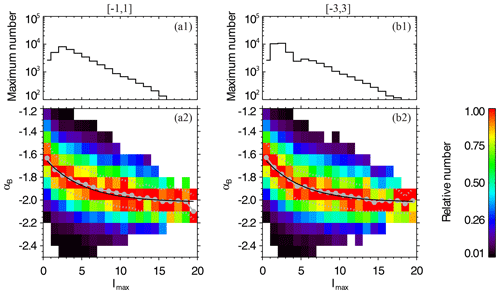Figure 12Panels (a1) and (a2) and panels (b1) and (b2) are plotted in the same format as Fig. 6 but for the respective PVI thresholds of $\left[-\mathrm{1},\mathrm{1}\right]$ and $\left[-\mathrm{3},\mathrm{3}\right]$ for identifying an intermittent interval. The black curves in panels (a2) and (b2) are both the exponential function ${\mathit{\alpha }}_{B}=\mathrm{0.4}\mathrm{exp}\left(-{I}_{\mathrm{max}}/\mathrm{5}\right)-\mathrm{2.02}$, which is adopted from Fig. 6.

5 Conclusions

In this paper, we present, for the first time, the analytical relation between the magnetic spectral index αB in the inertial range and the level of intermittency Imax at the timescale of τ=24 s in the slow solar wind. Data from Wind spacecraft observations between 2005 and 2013 are used for analysis. We preliminarily examine 56 398 intermittent structures using the criterion $|{\mathrm{PVI}}_{i}|>\mathrm{2}$ ($i=x,y,$ or z), with tB and tE being the beginning and ending instants of a structure, respectively. However, for more than half of the structures, the maximum I (Imax) during [tB,tE] (as marked by the two vertical dotted lines in Fig. 2) is not the maximum I during the corresponding plotted interval [tB−150 s, tE+150 s] (the whole plotted interval in Fig. 2). This means that, outside of [tB,tE], some other structures exist with an even higher level of intermittency during the interval [tB−150 s, tE+150 s]. We eliminate these kinds of intervals, during which the energy of the fluctuations is not dominated by the intermittent structure embedded in the center of it. In this way, we avoid the duplicate selection of the cases and also guarantee that both the intermittency level Imax and the magnetic spectral index αB are closely related to the intermittent structure embedded in the middle of each interval. We then obtain 25 912 intermittent intervals. Subsequently, the cases with a higher fitting error of the magnetic power spectra (${\mathrm{\Delta }}_{{\mathit{\alpha }}_{B}}/{\mathit{\alpha }}_{B}>\mathrm{5}\phantom{\rule{0.125em}{0ex}}\mathit{%}$) are eliminated, and 24 886 intermittent intervals are reserved for the statistical analysis.

Finally, we select 24 886 intermittent intervals and 17 386 quiet intervals from 46 slow-wind streams. Each intermittent interval lasts about 5–6 min with a dominant intermittent structure embedded in the center of it. The maximum I (Imax) of an intermittent structure is recorded as the intermittency level of the corresponding interval. The magnetic trace power spectrum of each interval is obtained by performing FFT on the high-resolution magnetic field data with $\mathrm{\Delta }{t}_{\mathrm{H}}=\mathrm{1}/\mathrm{11}$ s, and it is then linearly interpolated onto a uniformly spaced grid in the log–log space. The magnetic spectral index αB is obtained by performing a least-squares fit on the interpolated spectrum between 0.01 and 0.3 Hz in the inertial range. The selected intervals all have relatively low fitting errors (${\mathrm{\Delta }}_{{\mathit{\alpha }}_{B}}/{\mathit{\alpha }}_{B}\le \mathrm{5}\phantom{\rule{0.125em}{0ex}}\mathit{%}$), indicating that their magnetic power spectra have a good power-law shape.

The observed variation in the averaged spectral index αB as a function of the intermittency level Imax is presented in Fig. 6b as solid gray circles. When Imax increases from 0.5 to 15.5, the magnetic power spectrum gets steeper and the averaged magnetic spectral index αB decreases from $-{\mathrm{1.63}}_{-\mathrm{0.12}}^{+\mathrm{0.09}}$ to $-{\mathrm{1.99}}_{-\mathrm{0.11}}^{+\mathrm{0.09}}$. We also find that the averaged magnetic spectral index αB changes more quickly at Imax≤3.5 than at $\mathrm{3.5}<{I}_{\mathrm{max}}\le \mathrm{15.5}$. When Imax gets larger, the magnetic spectral index stops decreasing and remains almost constant at ${\mathit{\alpha }}_{B}\approx -\mathrm{2}$. However, the dependence of the magnetic spectral index on the magnetic compressibility seems not to be significant, as shown in Figs. 7 and 8.

According to the observational result, an empirical relation is built up between the magnetic spectral index αB and the intermittency level Imax as ${\mathit{\alpha }}_{B}=\mathrm{0.4}\mathrm{exp}\left(-{I}_{\mathrm{max}}/\mathrm{5}\right)-\mathrm{2.02}$. The empirical relation is illustrated using the black curve in Fig. 6b. It gives the continuous variation in the magnetic spectral index αB as a function of the intermittency level Imax. This relation will help people to easily estimate the contribution of the intermittency level to the magnetic spectral index, which implies the nature of the cascading process happening in the turbulence. It also supplies an observational constraint for numerical studies related to intermittency and spectral analysis of the solar wind turbulence. Moreover, from the aspect of theoretical study, the relation will help improve turbulence theories that contains intermittent structures.

We also check the sensitivity of the results based on the choice of the threshold for identifying an intermittent interval. The threshold is changed from the original PVI range of $\left[-\mathrm{2},\mathrm{2}\right]$ into two new respective ranges of $\left[-\mathrm{1},\mathrm{1}\right]$ and $\left[-\mathrm{3},\mathrm{3}\right]$. The results are shown in Fig. 12. Figure 12a1 and a2 correspond to the threshold of $\left[-\mathrm{1},\mathrm{1}\right]$ for identifying an intermittent interval, whereas Fig. 12b1 and b2 correspond to the threshold of $\left[-\mathrm{3},\mathrm{3}\right]$. Figure 12 is plotted in the same format as Fig. 6. The black curves in Fig. 12a2 and b2 are both the exponential function ${\mathit{\alpha }}_{B}=\mathrm{0.4}\mathrm{exp}\left(-{I}_{\mathrm{max}}/\mathrm{5}\right)-\mathrm{2.02}$, which is adopted from Fig. 6. It is found that the black curve obtained from the original threshold of $\left[-\mathrm{2},\mathrm{2}\right]$ can still match the new results well. Therefore, our result shown in Fig. 6 is robust and is not sensitive to the choice of the threshold for identifying intermittent intervals.

Moreover, mentioned that “The only change in the spectra for intervals containing a different number of discontinuities, or of discontinuities of differing magnitude, should be in the power levels, and not in the general spectral shape”. Based on the high-resolution data and sufficient samples observed by the Wind spacecraft, our results here provide observational evidence that the magnetic spectral shape (i.e., the spectral index in the inertial range) actually changes when the intermittency level of interval is different. Therefore, when researchers try to study the cascading process and evolution of the solar wind turbulence, it is very necessary to consider the effect of the intermittency level. In the future, we will also investigate the influence of the number of intermittent structures on the magnetic spectral shape. Additionally, it would also be interesting to establish the physical nature of the intermittent structures found in the slow-wind streams and to compare the result with those in the fast-wind streams .

Data availability

The Wind data are available through CDAWeb (https://cdaweb.gsfc.nasa.gov/; ). The magnetic field data used in this work include 3 s resolution (WI_H0_MFI) and high-resolution (WI_H2_MFI) data measured by magnetic field investigation between 2005 and 2013. The plasma data used here include 3 s resolution ion moments (WI_PM_3DP) measured using a 3D plasma analyzer between 2005 and 2013.

Author contributions

XW was primarily responsible for data analysis and for writing the article. XF also participated in data analysis. YW, HW, and LZ participated in the discussion and interpretation of the results and edited the manuscript.

Competing interests

The contact author has declared that none of the authors has any competing interests.

Disclaimer

Publisher’s note: Copernicus Publications remains neutral with regard to jurisdictional claims in published maps and institutional affiliations.

Acknowledgements

This work at Beihang University is supported by the National Natural Science Foundation of China (under contract nos. 41874199, 41974198, and 41504130). Xin Wang is also supported by the Fundamental Research Funds for the Central Universities of China (grant nos. KG16152401 and KG16159701). Moreover, this work received support from the B-type Strategic Priority Program of the Chinese Academy of Sciences (grant no. XDB41000000) and the pre-research projects on Civil Aerospace Technologies (grant nos. D020103 and D020105) funded by China's National Space Administration (CNSA).

Financial support

This research has been supported by the National Natural Science Foundation of China (grant nos. 41874199, 41974198, and 41504130), the Fundamental Research Funds for the Central Universities of China (grant nos. KG16152401 and KG16159701), the Btype Strategic Priority Program of the Chinese Academy of Sciences (grant no. XDB41000000), and the pre-research projects on Civil Aerospace Technologies (grant nos. D020103 and D020105) funded by China's National Space Administration (CNSA).

Review statement

This paper was edited by Georgios Balasis and reviewed by Joseph Borovsky and one anonymous referee.

References

Alberti, T., Laurenza, M., Consolini, G., Milillo, A., Marcucci, M. F., Carbone, V., and Bale, S. D.: On the Scaling Properties of Magnetic-field Fluctuations through the Inner Heliosphere, Astrophys. J., 902, 84–91, https://doi.org/10.3847/1538-4357/abb3d2, 2020. a

Bavassano, B., Dobrowolny, M., Fanfoni, G., Mariani, F., and Ness, N. F.: Statistical Properties of Magnetohydrodynamic Fluctuations Associated with High Speed Streams from HELIOS-2 Observations, Sol. Phys., 78, 373–384, https://doi.org/10.1007/BF00151617, 1982. a

Boldyrev, S., Nordlund, Å., and Padoan, P.: Scaling Relations of Supersonic Turbulence in Star-forming Molecular Clouds, Astrophys. J., 573, 678–684, https://doi.org/10.1086/340758, 2002. a

Borovsky, J. E.: Flux tube texture of the solar wind: Strands of the magnetic carpet at 1 AU?, J. Geophys. Res.-Space, 113, A08110, https://doi.org/10.1029/2007JA012684, 2008. a

Borovsky, J. E.: Contribution of Strong Discontinuities to the Power Spectrum of the Solar Wind, Phys. Rev. Lett., 105, 111102, https://doi.org/10.1103/PhysRevLett.105.111102, 2010. a, b, c

Borovsky, J. E.: The velocity and magnetic field fluctuations of the solar wind at 1 AU: Statistical analysis of Fourier spectra and correlations with plasma properties, J. Geophys. Res.-Space, 117, A05104, https://doi.org/10.1029/2011JA017499, 2012. a, b, c, d, e, f

Borovsky, J. E. and Burkholder, B. L.: On the Fourier Contribution of Strong Current Sheets to the High-Frequency Magnetic Power SpectralDensity of the Solar Wind, J. Geophys. Res.-Space, 125, e27307, https://doi.org/10.1029/2019JA027307, 2020. a, b, c, d

Borovsky, J. E. and Denton, M. H.: No Evidence for Heating of the Solar Wind at Strong Current Sheets, Astrophys. J. Lett., 739, L61–L65, https://doi.org/10.1088/2041-8205/739/2/L61, 2011. a

Borovsky, J. E. and Podesta, J. J.: Exploring the effect of current sheet thickness on the high-frequency Fourier spectrum breakpoint of the solar wind, J. Geophys. Res.-Space, 120, 9256–9268, https://doi.org/10.1002/2015JA021622, 2015. a

Bruno, R. and Carbone, V.: The Solar Wind as a Turbulence Laboratory, Living Rev. Sol. Phys., 10, 2–208, https://doi.org/10.12942/lrsp-2013-2, 2013. a

Bruno, R., Carbone, V., Veltri, P., Pietropaolo, E., and Bavassano, B.: Identifying intermittency events in the solar wind, Planet. Space Sci., 49, 1201–1210, https://doi.org/10.1016/S0032-0633(01)00061-7, 2001. a

Bruno, R., Carbone, V., Sorriso-Valvo, L., and Bavassano, B.: On the role of coherent and stochastic fluctuations in the evolving solar wind MHD turbulence: Intermittency, in: Solar Wind Ten, edited by: Velli, M., Bruno, R., Malara, F., and Bucci, B., Vol. 679, American Institute of Physics Conference Series, American Institute of Physics, https://doi.org/10.1063/1.1618632, 2003. a

Burlaga, L. F.: Micro-Scale Structures in the Interplanetary Medium, Sol. Phys., 4, 67–92, https://doi.org/10.1007/BF00146999, 1968. a, b

Burlaga, L. F.: Directional Discontinuities in the Interplanetary Magnetic Field, Sol. Phys., 7, 54–71, https://doi.org/10.1007/BF00148406, 1969. a

Burlaga, L. F.: Multifractal structure of the interplanetary magnetic field: Voyager 2 observations near 25 AU, 1987–1988, Geophys. Res. Lett., 18, 69–72, https://doi.org/10.1029/90GL02596, 1991a. a

Burlaga, L. F.: Intermittent turbulence in the solar wind, J. Geophys. Res., 96, 5847–5851, https://doi.org/10.1029/91JA00087, 1991b. a

Burlaga, L. F., Ness, N. F., and Acuna, M. H.: Multiscale structure of magnetic fields in the heliosheath, J. Geophys. Res.-Space, 111, A09112, https://doi.org/10.1029/2006JA011850, 2006. a

Carbone, V.: Cascade model for intermittency in fully developed magnetohydrodynamic turbulence, Phys. Rev. Lett., 71, 1546–1548, https://doi.org/10.1103/PhysRevLett.71.1546, 1993. a

Champeney, D. C.: Fourier transforms and their physical applications, Academic Press, London, 1973. a, b

Chandran, B. D. G., Schekochihin, A. A., and Mallet, A.: Intermittency and Alignment in Strong RMHD Turbulence, Astrophys. J., 807, 39, https://doi.org/10.1088/0004-637X/807/1/39, 2015. a

Chen, C., Mallet, A., Yousef, T., Schekochihin, A., and Horbury, T.: Anisotropy of Alfvénic turbulence in the solar wind and numerical simulations, Mon. Not. R. Astron. Soc., 415, 3219–3226, https://doi.org/10.1111/j.1365-2966.2011.18933.x, 2011. a, b

Chen, C. H. K., Bale, S. D., Bonnell, J. W., Borovikov, D., Bowen, T. A., Burgess, D., Case, A. W., Chandran, B. D. G., de Wit, T. D., Goetz, K., Harvey, P. R., Kasper, J. C., Klein, K. G., Korreck, K. E., Larson, D., Livi, R., MacDowall, R. J., Malaspina, D. M., Mallet, A., McManus, M. D., Moncuquet, M., Pulupa, M., Stevens, M. L., and Whittlesey, P.: The Evolution and Role of Solar Wind Turbulence in the Inner Heliosphere, Astrophys. J. Suppl. Ser., 246, 53–62, https://doi.org/10.3847/1538-4365/ab60a3, 2020. a

Cuesta, M. E., Parashar, T. N., Chhiber, R., and Matthaeus, W. H.: Intermittency in the Expanding Solar Wind: Observations from Parker Solar Probe (0.16 AU), Helios 1 (0.3–1 AU), and Voyager 1 (1–10 AU), Astrophys. J. Suppl. Ser., 259, 23–38, https://doi.org/10.3847/1538-4365/ac45fa, 2022. a

Dallas, V. and Alexakis, A.: Origins of the k−2 spectrum in decaying Taylor-Green magnetohydrodynamic turbulent flows, Phys. Rev. E, 88, 053014, https://doi.org/10.1103/PhysRevE.88.053014, 2013. a, b

Dudok de Wit, T.: Can high-order moments be meaningfully estimated from experimental turbulence measurements?, Phys. Rev. E, 70, 055302, https://doi.org/10.1103/PhysRevE.70.055302, 2004. a

Forman, M. A., Wicks, R. T., and Horbury, T. S.: Detailed Fit of “Critical Balance” Theory to Solar Wind Turbulence Measurements, Astrophys. J., 733, 76–83, https://doi.org/10.1088/0004-637X/733/2/76, 2011. a, b

Frisch, U.: Turbulence. The legacy of A.N. Kolmogorov, Cambridge University Press, Cambridge, 1995. a

Greco, A., Chuychai, P., Matthaeus, W. H., Servidio, S., and Dmitruk, P.: Intermittent MHD structures and classical discontinuities, Geophys. Res. Lett., 35, L19111, https://doi.org/10.1029/2008GL035454, 2008. a

Halsey, T. C., Jensen, M. H., Kadanoff, L. P., Procaccia, I., and Shraiman, B. I.: Fractal measures and their singularities: The characterization of strange sets, Phys. Rev. A, 33, 1141–1151, https://doi.org/10.1103/PhysRevA.33.1141, 1986. a

Horbury, T. S., Burgess, D., Fränz, M., and Owen, C. J.: Three spacecraft observations of solar wind discontinuities, Geophys. Res. Lett., 28, 677–680, https://doi.org/10.1029/2000GL000121, 2001. a

Horbury, T. S., Forman, M., and Oughton, S.: Anisotropic Scaling of Magnetohydrodynamic Turbulence, Phys. Rev. Lett., 101, 175005, https://doi.org/10.1103/PhysRevLett.101.175005, 2008. a, b

Knetter, T., Neubauer, F. M., Horbury, T., and Balogh, A.: Four-point discontinuity observations using Cluster magnetic field data: A statistical survey, J. Geophys. Res.-Space, 109, A06102, https://doi.org/10.1029/2003JA010099, 2004. a

Kolmogorov, A.: The Local Structure of Turbulence in Incompressible Viscous Fluid for Very Large Reynolds' Numbers, Akademiia Nauk SSSR Doklady, 30, 301–305, 1941. a

Lepping, R. P., Acũna, M. H., Burlaga, L. F., Farrell, W. M., Slavin, J. A., Schatten, K. H., Mariani, F., Ness, N. F., Neubauer, F. M., Whang, Y. C., Byrnes, J. B., Kennon, R. S., Panetta, P. V., Scheifele, J., and Worley, E. M.: The Wind Magnetic Field Investigation, Space Sci. Rev., 71, 207–229, https://doi.org/10.1007/BF00751330, 1995. a

Li, G., Miao, B., Hu, Q., and Qin, G.: Effect of Current Sheets on the Solar Wind Magnetic Field Power Spectrum from the Ulysses Observation: From Kraichnan to Kolmogorov Scaling, Phys. Rev. Lett., 106, 125001, https://doi.org/10.1103/PhysRevLett.106.125001, 2011. a, b, c, d, e, f

Lin, R. P., Anderson, K. A., Ashford, S., Carlson, C., Curtis, D., Ergun, R., Larson, D., McFadden, J., McCarthy, M., Parks, G. K., Rème, H., Bosqued, J. M., Coutelier, J., Cotin, F., D'Uston, C., Wenzel, K.-P., Sanderson, T. R., Henrion, J., Ronnet, J. C., and Paschmann, G.: A Three-Dimensional Plasma and Energetic Particle Investigation for the Wind Spacecraft, Space Sci. Rev., 71, 125–153, https://doi.org/10.1007/BF00751328, 1995. a

Liu, Y. Y., Fu, H. S., Liu, C. M., Wang, Z., Escoubet, P., Hwang, K. J., Burch, J. L., and Giles, B. L.: Parallel Electron Heating by Tangential Discontinuity in the Turbulent Magnetosheath, Astrophys. J. Lett., 877, L16–L21, https://doi.org/10.3847/2041-8213/ab1fe6, 2019. a

Liu, Y. Y., Fu, H. S., Cao, J. B., Liu, C. M., Wang, Z., Guo, Z. Z., Xu, Y., Bale, S. D., and Kasper, J. C.: Characteristics of Interplanetary Discontinuities in the Inner Heliosphere Revealed by Parker Solar Probe, Astrophys. J., 916, 65–72, https://doi.org/10.3847/1538-4357/ac06a1, 2021. a

Luo, Q. Y. and Wu, D. J.: Observations of Anisotropic Scaling of Solar Wind Turbulence, Astrophys. J. Lett., 714, L138–L141, https://doi.org/10.1088/2041-8205/714/1/L138, 2010. a, b

Macek, W. M. and Wawrzaszek, A.: Evolution of asymmetric multifractal scaling of solar wind turbulence in the outer heliosphere, J. Geophys. Res.-Space, 114, A03108, https://doi.org/10.1029/2008JA013795, 2009. a

Macek, W. M., Bruno, R., and Consolini, G.: Generalized dimensions for fluctuations in the solar wind, Phys. Rev. E, 72, 017202, https://doi.org/10.1103/PhysRevE.72.017202, 2005. a

Macek, W. M., Wawrzaszek, A., and Carbone, V.: Observation of the multifractal spectrum in the heliosphere and the heliosheath by Voyager 1 and 2, J. Geophys. Res.-Space, 117, A12101, https://doi.org/10.1029/2012JA018129, 2012. a

Marsch, E. and Tu, C. Y.: Non-Gaussian probability distributions of solar wind fluctuations, Ann. Geophys., 12, 1127–1138, https://doi.org/10.1007/s00585-994-1127-8, 1994. a, b

Marsch, E. and Tu, C. Y.: Intermittency, non-Gaussian statistics and fractal scaling of MHD fluctuations in the solar wind, Nonlin. Process. Geophys., 4, 101–124, https://doi.org/10.5194/npg-4-101-1997, 1997. a

Marsch, E., Tu, C. Y., and Rosenbauer, H.: Multifractal scaling of the kinetic energy flux in solar wind turbulence, Ann. Geophys., 14, 259–269, https://doi.org/10.1007/s00585-996-0259-4, 1996. a

Miao, B., Peng, B., and Li, G.: Current sheets from Ulysses observation, Ann. Geophys., 29, 237–249, https://doi.org/10.5194/angeo-29-237-2011, 2011. a

Neugebauer, M., Clay, D. R., Goldstein, B. E., Tsurutani, B. T., and Zwickl, R. D.: A reexamination of rotational and tangential discontinuities in the solar wind, J. Geophys. Res., 89, 5395–5408, https://doi.org/10.1029/JA089iA07p05395, 1984. a

NASA: Coordinated Data Analysis Web (CDAWeb), NASA [data set], https://cdaweb.gsfc.nasa.gov/, last access: 27 March 2023. a

Osman, K. T., Matthaeus, W. H., Greco, A., and Servidio, S.: Evidence for Inhomogeneous Heating in the Solar Wind, Astrophys. J. Lett., 727, L11–L15, https://doi.org/10.1088/2041-8205/727/1/L11, 2011. a, b

Osman, K. T., Matthaeus, W. H., Hnat, B., and Chapman, S. C.: Kinetic Signatures and Intermittent Turbulence in the Solar Wind Plasma, Phys. Rev. Lett., 108, 261103, https://doi.org/10.1103/PhysRevLett.108.261103, 2012a. a

Osman, K. T., Matthaeus, W. H., Wan, M., and Rappazzo, A. F.: Intermittency and Local Heating in the Solar Wind, Phys. Rev. Lett., 108, 261102, https://doi.org/10.1103/PhysRevLett.108.261102, 2012b. a

Osman, K. T., Matthaeus, W. H., Gosling, J. T., Greco, A., Servidio, S., Hnat, B., Chapman, S. C., and Phan, T. D.: Magnetic Reconnection and Intermittent Turbulence in the Solar Wind, Phys. Rev. Lett., 112, 215002, https://doi.org/10.1103/PhysRevLett.112.215002, 2014. a

Paladin, G. and Vulpiani, A.: Anomalous scaling laws in multifractal objects, Phys. Rep., 156, 147–225, https://doi.org/10.1016/0370-1573(87)90110-4, 1987. a

Parashar, T. N., Shay, M. A., Cassak, P. A., and Matthaeus, W. H.: Kinetic dissipation and anisotropic heating in a turbulent collisionless plasma, Phys. Plasmas, 16, 032310, https://doi.org/10.1063/1.3094062, 2009. a

Podesta, J. J.: Dependence of Solar-Wind Power Spectra on the Direction of the Local Mean Magnetic Field, Astrophys. J., 698, 986–999, https://doi.org/10.1088/0004-637X/698/2/986, 2009. a, b

Podesta, J. J.: Spectra that behave like power-laws are not necessarily power-laws, Adv. Space Res., 57, 1127–1132, https://doi.org/10.1016/j.asr.2015.12.020, 2016. a, b, c

Podesta, J. J. and Borovsky, J. E.: Relationship between the durations of jumps in solar wind time series and the frequency of the spectral break, J. Geophys. Res.-Space, 121, 1817–1838, https://doi.org/10.1002/2015JA021987, 2016. a

Politano, H. and Pouquet, A.: Model of intermittency in magnetohydrodynamic turbulence, Phys. Rev. E, 52, 636–641, https://doi.org/10.1103/PhysRevE.52.636, 1995. a

Riazantseva, M. O., Zastenker, G. N., Richardson, J. D., and Eiges, P. E.: Sharp boundaries of small- and middle-scale solar wind structures, J. Geophys. Res.-Space, 110, A12110, https://doi.org/10.1029/2005JA011307, 2005. a

Roberts, D. A. and Goldstein, M. L.: Spectral signatures of jumps and turbulence in interplanetary speed and magnetic field data, J. Geophys. Res., 92, 10 105–10 110, https://doi.org/10.1029/JA092iA09p10105, 1987. a, b

Salem, C., Mangeney, A., Bale, S. D., Veltri, P., and Bruno, R.: Anomalous scaling and the role of intermittency in solar wind MHD turbulence: new insights, in: Turbulence and Nonlinear Processes in Astrophysical Plasmas, edited by: Shaikh, D. and Zank, G. P., Vol. 932, American Institute of Physics Conference Series, American Institute of Physics, 75–82, https://doi.org/10.1063/1.2778948, 2007. a, b, c

Salem, C., Mangeney, A., Bale, S. D., and Veltri, P.: Solar Wind Magnetohydrodynamics Turbulence: Anomalous Scaling and Role of Intermittency, Astrophys. J., 702, 537–553, https://doi.org/10.1088/0004-637X/702/1/537, 2009. a, b, c, d

Sari, J. W. and Ness, N. F.: Power Spectra of the Interplanetary Magnetic Field, Sol. Phys., 8, 155–165, https://doi.org/10.1007/BF00150667, 1969. a, b, c, d

Servidio, S., Valentini, F., Califano, F., and Veltri, P.: Local Kinetic Effects in Two-Dimensional Plasma Turbulence, Phys. Rev. Lett., 108, 045001, https://doi.org/10.1103/PhysRevLett.108.045001, 2012. a, b

She, Z.-S. and Leveque, E.: Universal scaling laws in fully developed turbulence, Phys. Rev. Lett., 72, 336–339, https://doi.org/10.1103/PhysRevLett.72.336, 1994. a

Sioulas, N., Huang, Z., Velli, M., Chhiber, R., Cuesta, M. E., Shi, C., Matthaeus, W. H., Bandyopadhyay, R., Vlahos, L., Bowen, T. A., Qudsi, R. A., Bale, S. D., Owen, C. J., Louarn, P., Fedorov, A., Maksimović, M., Stevens, M. L., Case, A., Kasper, J., Larson, D., Pulupa, M., and Livi, R.: Magnetic Field Intermittency in the Solar Wind: Parker Solar Probe and SolO Observations Ranging from the Alfvén Region up to 1 AU, Astrophys. J., 934, 143–159, https://doi.org/10.3847/1538-4357/ac7aa2, 2022. a

Siscoe, G. L., Davis, L., J., Coleman, P. J., J., Smith, E. J., and Jones, D. E.: Power spectra and discontinuities of the interplanetary magnetic field: Mariner 4, J. Geophys. Res., 73, 61–82, https://doi.org/10.1029/JA073i001p00061, 1968. a, b

Sonnerup, B. U. O. and Cahill Jr., L. J.: Magnetopause Structure and Attitude from Explorer 12 Observations, J. Geophys. Res., 72, 171–183, https://doi.org/10.1029/JZ072i001p00171, 1967. a

Sorriso-Valvo, L., Carbone, F., Leonardis, E., Chen, C. H. K., Šafránková, J., and Němeček, Z.: Multifractal analysis of high resolution solar wind proton density measurements, Adv. Space Res., 59, 1642–1651, https://doi.org/10.1016/j.asr.2016.12.024, 2017. a, b

Telloni, D., Carbone, F., Bruno, R., Sorriso-Valvo, L., Zank, G. P., Adhikari, L., and Hunana, P.: No Evidence for Critical Balance in Field-aligned Alfvénic Solar Wind Turbulence, Astrophys. J., 887, 160–166, https://doi.org/10.3847/1538-4357/ab517b, 2019. a, b

Tsurutani, B. T. and Ho, C. M.: A review of discontinuities and Alfvén waves in interplanetary space: Ulysses results, Rev. Geophys., 37, 517–524, https://doi.org/10.1029/1999RG900010, 1999. a

Tu, C. Y. and Marsch, E.: A case study of very low cross-helicity fluctuations in the solar wind., Ann. Geophys., 9, 319–332, 1991. a

Tu, C.-Y. and Marsch, E.: MHD structures, waves and turbulence in the solar wind: Observations and theories, Space Sci. Rev., 73, 1–210, https://doi.org/10.1007/BF00748891, 1995. a

Veltri, P.: MHD turbulence in the solar wind: self-similarity, intermittency and coherent structures, Plasma Phys. Controll. Fusion, 41, A787–A795, https://doi.org/10.1088/0741-3335/41/3A/071, 1999. a

Veltri, P. and Mangeney, A.: Scaling laws and intermittent structures in solar wind MHD turbulence, in: Solar Wind Nine, edited by: Habbal, S. R., Esser, R., Hollweg, J. V., and Isenberg, P. A., Vol. 471, American Institute of Physics Conference Series, American Institute of Physics, 543–546, https://doi.org/10.1063/1.58809, 1999. a, b, c

Wan, M., Matthaeus, W. H., Karimabadi, H., Roytershteyn, V., Shay, M., Wu, P., Daughton, W., Loring, B., and Chapman, S. C.: Intermittent Dissipation at Kinetic Scales in Collisionless Plasma Turbulence, Phys. Rev. Lett., 109, 195001, https://doi.org/10.1103/PhysRevLett.109.195001, 2012. a

Wang, X., Tu, C., He, J., Marsch, E., and Wang, L.: On Intermittent Turbulence Heating of the Solar Wind: Differences between Tangential and Rotational Discontinuities, Astrophys. J. Lett., 772, L14–L20, https://doi.org/10.1088/2041-8205/772/2/L14, 2013. a, b, c, d, e, f

Wang, X., Tu, C., He, J., Marsch, E., and Wang, L.: The Influence of Intermittency on the Spectral Anisotropy of Solar Wind Turbulence, Astrophys. J. Lett., 783, L9–L15, https://doi.org/10.1088/2041-8205/783/1/L9, 2014. a, b

Wang, X., Tu, C., He, J., Marsch, E., Wang, L., and Salem, C.: The Spectral Features of Low-amplitude Magnetic Fluctuations in the Solar Wind and Their Comparison with Moderate-amplitude Fluctuations, Astrophys. J. Lett., 810, L21–L27, https://doi.org/10.1088/2041-8205/810/2/L21, 2015. a, b

Wang, X., Tu, C., and He, J.: Fluctuation Amplitudes of Magnetic-field Directional Turnings and Magnetic-velocity Alignment Structures in the Solar Wind, Astrophys. J., 903, 72–82, https://doi.org/10.3847/1538-4357/abb883, 2020. a, b, c

Wicks, R. T., Horbury, T. S., Chen, C. H. K., and Schekochihin, A. A.: Anisotropy of Imbalanced Alfvénic Turbulence in Fast Solar Wind, Phys. Rev. Lett., 106, 045001, https://doi.org/10.1103/PhysRevLett.106.045001, 2011. a, b

Wicks, R. T., Mallet, A., Horbury, T. S., Chen, C. H. K., Schekochihin, A. A., and Mitchell, J. J.: Alignment and Scaling of Large-Scale Fluctuations in the Solar Wind, Phys. Rev. Lett., 110, 025003-1, https://doi.org/10.1103/PhysRevLett.110.025003, 2013a. a

Wu, H., Tu, C., Wang, X., He, J., Yang, L., and Wang, L.: Isotropic Scaling Features Measured Locally in the Solar Wind Turbulence with Stationary Background Field, Astrophys. J., 892, 138–147, https://doi.org/10.3847/1538-4357/ab7b72, 2020.  a

Wu, H., Tu, C., Wang, X., and Yang, L.: Large Amplitude Switchback Turbulence: Possible Magnetic Velocity Alignment Structures, Astrophys. J., 911, 73–79, https://doi.org/10.3847/1538-4357/abec6c, 2021. a

Yang, L., He, J., Tu, C., Li, S., Zhang, L., Wang, X., Marsch, E., and Wang, L.: Influence of Intermittency on the Quasi-perpendicular Scaling in Three-dimensional Magnetohydrodynamic Turbulence, Astrophys. J., 846, 49–58, https://doi.org/10.3847/1538-4357/aa7e7c, 2017. a

Zhang, L., He, J., Tu, C., Yang, L., Wang, X., Marsch, E., and Wang, L.: Occurrence Rates and Heating Effects of Tangential and Rotational Discontinuities as Obtained from Three-dimensional Simulation of Magnetohydrodynamic Turbulence, Astrophys. J. Lett., 804, L43–L49, https://doi.org/10.1088/2041-8205/804/2/L43, 2015. a

Zhou, Z., Xu, X., Zuo, P., Wang, Y., Xu, Q., Ye, Y., Wang, J., Wang, M., Chang, Q., Wang, X., and Luo, L.: Evidence for Plasma Heating at Thin Current Sheets in the Solar Wind, Astrophys. J. Lett., 924, L22–L27, https://doi.org/10.3847/2041-8213/ac4701, 2022. a